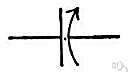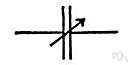# variable

(redirected from Endogenous variable)
Also found in: Thesaurus, Medical, Legal, Financial, Encyclopedia.

## var·i·a·ble

(vâr′ē-ə-bəl, văr′-)
1.
a. Likely to change or vary; subject to variation; changeable.
b. Inconstant; fickle.
2. Biology Tending to exhibit genetic variation or variation in a physical trait: geographically variable color patterns.
3. Mathematics Having no fixed quantitative value.
n.
1. Something that varies or is prone to variation.
2. Astronomy A variable star.
3. Mathematics
a. A quantity capable of assuming any of a set of values.
b. A symbol representing such a quantity. For example, in the expression a2 + b2 = c2, a,b, and c are variables.

var′i·a·ble·ness n.

## variable

(ˈvɛərɪəbəl)
1. liable to or capable of change: variable weather.
2. (of behaviour, opinions, emotions, etc) lacking constancy; fickle
3. (Mathematics) maths having a range of possible values
4. (Biology) (of a species, characteristic, etc) liable to deviate from the established type
5. (Physical Geography) (of a wind) varying its direction and intensity
6. (Electrical Engineering) (of an electrical component or device) designed so that a characteristic property, such as resistance, can be varied: variable capacitor.
n
7. something that is subject to variation
8. (Mathematics) maths
a. an expression that can be assigned any of a set of values
b. a symbol, esp x, y, or z, representing an unspecified member of a class of objects, numbers, etc. See also dependent variable, independent variable
9. (Logic) logic a symbol, esp x, y, z, representing any member of a class of entities
10. (Computer Science) computing a named unit of storage that can be changed to any of a set of specified values during execution of a program
11. (Celestial Objects) astronomy See variable star
12. (Physical Geography) a variable wind
13. (Physical Geography) (plural) a region where variable winds occur
[C14: from Latin variābilis changeable, from variāre to diversify]
ˌvariaˈbility, ˈvariableness n

## var•i•a•ble

(ˈvɛər i ə bəl)

1. apt to vary; changeable.
2. capable of being varied.
3. inconstant; fickle.
4. having much variation or diversity.
5. deviating from the usual type, as a species.
6. (of a star) changing in brightness.
7. (of wind) tending to change in direction.
8. having the characteristics of a variable.
n.
9. something that may vary.
10.
a. a quantity or function that may assume any given value or set of values.
b. a symbol that represents this.
11. a symbol in logic for an unspecified member of a class of things or statements.
12. a shifting wind.
[1350–1400; < Late Latin]
var`i•a•bil′i•ty, var′i•a•ble•ness, n.

## var·i·a·ble

(vâr′ē-ə-bəl)
1. A mathematical quantity capable of assuming any of a set of values, such as x in the expression 3x + 2.
2. A factor or condition that is subject to change, especially one that is allowed to change in a scientific experiment to test a hypothesis. See more at control.

## variable

A condition, such as temperature, concentration and pressure, which can be changed in a chemical reaction.
ThesaurusAntonymsRelated WordsSynonymsLegend:
 Noun 1 variable - something that is likely to vary; something that is subject to variation; "the weather is one variable to be considered"thing - a separate and self-contained entity 2variable - a quantity that can assume any of a set of valuesvariable quantityquantity - the concept that something has a magnitude and can be represented in mathematical expressions by a constant or a variableargument - a variable in a logical or mathematical expression whose value determines the dependent variable; if f(x)=y, x is the independent variableexperimental variable, independent variable - (statistics) a variable whose values are independent of changes in the values of other variablescorrelate, correlative - either of two or more related or complementary variablesdegree of freedom - (statistics) an unrestricted variable in a frequency distributiondependent variable - (statistics) a variable in a logical or mathematical expression whose value depends on the independent variable; "if f(x)=y, y is the dependent variable"predictor variable - a variable that can be used to predict the value of another variable (as in statistical regression)infinitesimal - (mathematics) a variable that has zero as its limitchance variable, random variable, stochastic variable, variate, variant - a variable quantity that is randomscalar - a variable quantity that cannot be resolved into componentstensor - a generalization of the concept of a vectorvector - a variable quantity that can be resolved into components 3variable - a star that varies noticeably in brightnessvariable starstar - (astronomy) a celestial body of hot gases that radiates energy derived from thermonuclear reactions in the interior 4variable - a symbol (like x or y) that is used in mathematical or logical expressions to represent a variable quantitysymbol - an arbitrary sign (written or printed) that has acquired a conventional significanceplaceholder - a symbol in a logical or mathematical expression that can be replaced by the name of any member of specified setunknown quantity, unknown - a variable whose values are solutions of an equation Adj. 1 variable - liable to or capable of change; "rainfall in the tropics is notoriously variable"; "variable winds"; "variable expenses"inconsistent - displaying a lack of consistency; "inconsistent statements cannot both be true at the same time"; "inconsistent with the roadmap"inconstant - likely to change frequently often without apparent or cogent reason; variable; "inconstant affections"; "an inconstant lover"; "swear not by...the inconstant moon"- Shakespeareinvariable - not liable to or capable of change; "an invariable temperature"; "an invariable rule"; "his invariable courtesy" 2variable - marked by diversity or difference; "the varying angles of roof slope"; "nature is infinitely variable"varyingvaried - characterized by variety; "immigrants' varied ethnic and religious traditions"; "his work is interesting and varied" 3 variable - (used of a device) designed so that a property (as e.g. light) can be varied; "a variable capacitor"; "variable filters in front of the mercury xenon lights"adaptable - capable of adapting (of becoming or being made suitable) to a particular situation or use; "to succeed one must be adaptable"; "the frame was adaptable to cloth bolts of different widths"

## variable

adjective Weather conditions are variable and change from day to day.

## variable

1. Capable of or liable to change:
Archaic: various.
2. Following no predictable pattern:
3. Lacking consistency or regularity in quality or performance:
Translations
مُتَغَيِّرمُتَغَيِّر، كَمِيَّة مُتَغَيِّرَهمُتَقَلِّبمُتَنَوِّع، مُخْتَلِف
proměnlivýproměnnáfaktorměnitelnýmožná změna
variabel
muutuja
vaihtelevamuuttuja
promjenjiv
változóváltoztatható
breytabreytanlegur, stillanlegurbreytilegur, óstöîugur

변하기 쉬운
meniteľnýmožná výmena
avvikandeparametervariabelvarierande
เปลี่ยนแปลงได้ตลอดเวลา
değişkendeğişken nicelikkararsızdeğişebilendeğişik
có thể thay đổi

## variable

[ˈvɛərɪəbl]
A. ADJ (gen) → variable; [person] →
variable costs
B. N

## variable

[ˈvɛəriəbəl]
[amount, content, quality, rainfall, weather, rate, speed] → variable
a variable rate of interest
[mood] → changeant(e)
n
(gen) (= factor) →
(MATHEMATICS)

## variable

(= likely to vary) (Math) → ; (Biol) → variabel; weather, mood; infinitely variable (Tech) → stufenlos; variable winds pl; his work is very variableer arbeitet sehr unterschiedlich
speedregulierbar; salary levelflexibel; the height of the seat is variabledie Höhe des Sitzes kann reguliert werden
n (Chem, Math, Phys, Comput) → Variable f; (fig also); dependent/independent variable (Math) →

## variable

[ˈvɛərɪəbl]
1. adj (output, performance) → non costante; (weather, wind) → variabile; (mood) → mutevole
2. n (Math) →

## vary

(ˈveəri) verb
to make, be or become different. These apples vary in size from small to medium.
1. that may be varied. The machine works at a variable speed.
2. (of eg winds, weather etc) liable or likely to change. British weather is very variable.
noun
something that varies, eg in quantity, value, effect etc. Have you taken all the variables into account in your calculations?
ˌvariaˈbilityplural variaˈbilities noun
ˌvariˈation noun
1. the extent to which a thing changes. In the desert there are great variations in temperature.
2. one of a series of musical elaborations made on a basic theme or melody. Brahms' variations on Haydn's `St Anthony's Chorale'.
He has had a very varied career.

## variable

proměnlivý variabel vaihteleva promjenjiv 変わりやすい 변하기 쉬운 varierande เปลี่ยนแปลงได้ตลอดเวลา có thể thay đổi

## var·i·a·ble

n. variable, factor que puede variar;
a. que puede cambiar.

## variable

adj & n variable f
References in periodicals archive ?
On the other hand, practice as latent endogenous variable contained four observed variables including abstinence, faithfulness, and condom use as well as practicing desirable health related behaviours.
With the absence of mediation role, differentiation advantage is served as the endogenous variable to the direct relationship with green service strategies (green employee empowerment and green physical evidence).
If the utility functions are interdependent, the endogenous variable for price and quantity in each equation should be significant.
Moreover, the path coefficients of each exogenous variable on endogenous variable were used to calculate the Total effect.
Crash-Per-Million-Miles is the endogenous variable in the SEM.
An endogenous variable is influenced by other variable(s) in the model.
The first regression used the ultimate endogenous variable, college GPA, as the dependent variable and the following five regressions used each of the previously identified factor constructs for dependent variables.
We then specify an endogenous dummy variable model, where participation in tourism related work is assumed to be endogenous variable.
Endogenous variable is a variable that is an inherent part of the system being studied and determined from within the system.
For instrumental variable estimation, parameter identification requires presence in the switching equation of at least one variable which is correlated with the endogenous variable, uncorrelated with error terms of the outcome equations, and does not affect the outcome variable.
This method requires that we identify an instrument which is correlated with the endogenous variable but not with the error term in the model.

Site: Follow: Share:
Open / Close